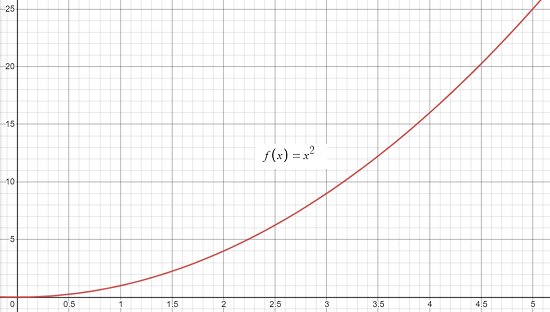# The Area Problem

Finding the area under the curve of a function is called the area problem. Geometric shapes like squares and rectangles, which have defined formulas, can be used to find curved areas which don’t have common formulas.

The area problem only works for continuous functions that are non-negative (i.e. above the x-axis).

## The Area Problem: Example

Example question: What is the area of the shape under the curve of f(x) = x2 on the closed interval [1, 5]?

Step 1: Graph the function (I used Desmos.com):We can see the “problem” here (and why it’s called the area problem); We don’t have an obvious formula for the shape created in the interval [1,5]. We could do some fandangling with an inverted ellipse (the shape looks like one quarter of an inverted one) but a much easier way is to approximate the area with rectangles.

Step 3: Draw the rectangles. We could draw any number of rectangles we like. For this example, we can get a reasonable approximation of the area (good enough for ordering tiles or carpet!) with 4 rectangles:Four rectangles, centered at the midpoint of each interval (1.5, 2.5, 3.5, & 4.5).

Step 4: Calculate the area of each rectangle. Calculate the area of each rectangle. There are two ways to do this: you could just look at the graph and ballpark the length and width of each rectangle. A better way is to use the function we’re given: x2:

 Rectangle # Length ( on x-axis) Height (on y-axis) A = L * H 1 1 f(1.5) = (1.5)2 = 2.25 1 * 2.25 = 2.25 2 1 f(2.5) = (2.5)2 = 6.25 1 * 6.25 = 6.25 3 1 f(3.5) = (3.5)2 = 12.25 1 * 12.25 = 12.25 4 1 f(4.5) = (4.5)2 = 20.25 1 * 20.25 = 20.25

Step 5: Add up the rectangle areas from Step 4.

2.25 + 6.25 + 12.25 + 20.25 = 41.
The area under the curve is approximately 41 units.

## Exact Solution

The solution of 41 is very close to the exact area (which we can get with a method called integration) of 124/3. To get the exact answer, we use definite integrals:

1. Go to Symbolab’s Calculator.
2. Click on the little grey boxes at the top and bottom of the integral symbol (∫) and type in 5 (At the top) and 1 (at the bottom). These are called integral bounds.3. Type in x2 in between the integral symbol and the “dx”. Then click the red “Go” button on the right.The exact solution is 124/3 ≈ 41.333.

## The Area Problem and Riemann Sums

In the above example, I used the midpoint of each interval to calculate the areas. I could also have used inscribed rectangles (under the curve) or circumscribed rectangles (above the curve). Calculation with all of these different sums are covered in the method of Riemann sums.

CITE THIS AS:
Stephanie Glen. "The Area Problem" From StatisticsHowTo.com: Elementary Statistics for the rest of us! https://www.statisticshowto.com/the-area-problem/